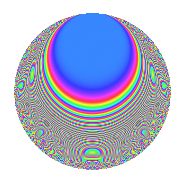# Properties

 Label 109.2.hLevel 109 Weight 2 Character orbit h Rep. character $$\chi_{109}(4,\cdot)$$ Character field $$\Q(\zeta_{18})$$ Dimension 48 Newform subspaces 1 Sturm bound 18 Trace bound 0

# Related objects

## Defining parameters

 Level: $$N$$ = $$109$$ Weight: $$k$$ = $$2$$ Character orbit: $$[\chi]$$ = 109.h (of order $$18$$ and degree $$6$$) Character conductor: $$\operatorname{cond}(\chi)$$ = $$109$$ Character field: $$\Q(\zeta_{18})$$ Newform subspaces: $$1$$ Sturm bound: $$18$$ Trace bound: $$0$$

## Dimensions

The following table gives the dimensions of various subspaces of $$M_{2}(109, [\chi])$$.

Total New Old
Modular forms 60 60 0
Cusp forms 48 48 0
Eisenstein series 12 12 0

## Trace form

 $$48q - 9q^{2} - 6q^{3} + 15q^{4} - 6q^{5} + 3q^{7} + 12q^{9} + O(q^{10})$$ $$48q - 9q^{2} - 6q^{3} + 15q^{4} - 6q^{5} + 3q^{7} + 12q^{9} - 9q^{10} + 9q^{11} - 3q^{12} - 15q^{14} - 12q^{15} - 3q^{16} - 9q^{17} - 69q^{18} - 9q^{19} - 42q^{20} + 15q^{21} + 12q^{22} - 18q^{23} + 60q^{24} - 18q^{25} - 12q^{26} - 45q^{27} - 54q^{28} + 33q^{29} - 33q^{30} - 18q^{31} + 9q^{32} + 3q^{34} + 18q^{35} + 105q^{36} + 15q^{37} - 33q^{38} - 6q^{39} - 12q^{40} - 36q^{42} + 9q^{43} + 66q^{44} - 6q^{45} - 60q^{46} - 6q^{47} + 57q^{48} + 39q^{49} + 87q^{50} - 48q^{51} - 36q^{52} + 15q^{53} + 90q^{54} - 9q^{55} + 3q^{56} + 15q^{57} - 27q^{58} - 6q^{59} - 33q^{60} + 78q^{61} + 36q^{62} + 72q^{63} + 126q^{64} - 54q^{65} + 9q^{66} + 54q^{67} - 3q^{69} + 39q^{70} + 15q^{71} - 99q^{72} - 21q^{73} + 24q^{74} - 30q^{75} + 81q^{77} + 27q^{78} - 126q^{79} + 45q^{80} - 75q^{81} - 3q^{82} - 21q^{83} - 63q^{84} - 6q^{85} - 189q^{86} + 114q^{87} + 12q^{88} + 3q^{89} + 225q^{90} - 72q^{91} - 27q^{92} + 33q^{93} - 42q^{94} - 39q^{95} - 99q^{96} + 51q^{97} - 150q^{98} - 93q^{99} + O(q^{100})$$

## Decomposition of $$S_{2}^{\mathrm{new}}(109, [\chi])$$ into newform subspaces

Label Dim. $$A$$ Field CM Traces $q$-expansion
$$a_2$$ $$a_3$$ $$a_5$$ $$a_7$$
109.2.h.a $$48$$ $$0.870$$ None $$-9$$ $$-6$$ $$-6$$ $$3$$

## Hecke Characteristic Polynomials

There are no characteristic polynomials of Hecke operators in the database